# VB.Net Operators: Arithmetic, Comparison, Logical with EXAMPLES

## What is an operator?

An operator refers to a symbol that instructs the compiler to perform a specific logical or mathematical manipulation. The operator performs the operation on the provided operands. Microsoft VB.Net comes with various types of operators. We will be discussing these in this tutorial.

In this tutorial, you will learn-

## Arithmetic Operators

You can use arithmetic operators to perform various mathematical operations in VB.NET. They include:

 Symbol Description ^ for raising an operand to the power of another operand + for adding two operands. – for subtracting the second operand from the first operand. * for multiplying both operands. / for dividing an operand against another. It returns a floating point result. \ for dividing an operand against another. It returns an integer result. MOD known as the modulus operator. It returns the remainder after division.

Let us demonstrate how to use these using an example:

Step 1) Create a new console application. To know this, visit our previous tutorial on Data Types and Variables.

Step 2) Add the following code:

```Module Module1

Sub Main()
Dim var_w As Integer = 11
Dim var_x As Integer = 5
Dim var_q As Integer = 2
Dim var_y As Integer
Dim var_z As Single

var_y = var_w + var_z
Console.WriteLine(" Result of 11 + 5 is {0} ", var_y)

var_y = var_w - var_x
Console.WriteLine(" Result of 11 - 5 is {0} ", var_y)

var_y = var_w * var_x
Console.WriteLine(" Result of 11 * 5 is {0} ", var_y)

var_z = var_w / var_x
Console.WriteLine(" Result of 11 / 5 is {0}", var_z)

var_y = var_w \ var_x
Console.WriteLine(" Result of 11 \ 5 is {0}", var_y)

var_y = var_w Mod var_x
Console.WriteLine(" Result of 11 MOD 5 is {0}", var_y)

var_y = var_x ^ var_x
Console.WriteLine(" Result of 5 ^ 5 is {0}", var_y)

End Sub

End Module
```

Step 3) Click the Start button to execute the code. You should get the following window: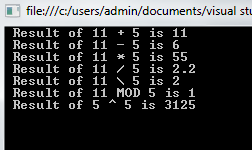Here is a screenshot of the code: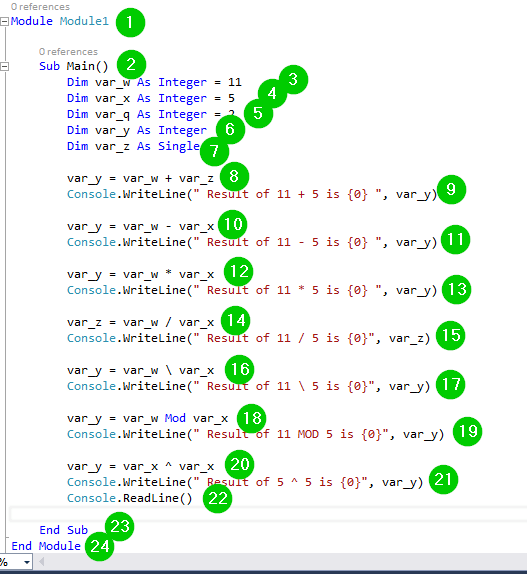Explanation of Code:

1. Creating a module named Module1.
2. Creating the main sub-procedure.
3. Creating an integer variable var_w with a value of 11.
4. Creating an integer variable var_x with a value of 5.
5. Creating an integer var_q with a value of 2.
6. Creating an integer var_y.
7. Creating an integer var_z.
8. Adding the values of variables var_w and var_z and assigning the result to variable var_y.
9. Printing the above result on the console.
10. Subtracting the value of variables var_x from the value of variable var_w and assigning the result to variable var_y.
11. Printing the above result on the console.
12. Multiplying the values of variables var_w and var_x and assigning the result to variable var_y.
13. Printing the above result on the console.
14. Dividing the value of variable var_w by the value of variable var_x and assigning the result to variable var_z.
15. Printing the above result on the console.
16. Dividing the value of variable var_w by the value of variable var_x and assigning the result to variable var_y.
17. Printing the above result on the console.
18. Getting the remainder after dividing the value of variable var_w by the value of variable var_x and assigning the result to variable var_y.
19. Printing the above result on the console.
20. Getting the value of variable var_x raised to the power of the same and assigning the result to variable var_y.
21. Printing the above result on the console.
22. To exit the console when the user presses any key.
23. Ending the subprocedure.
24. Ending the Module

## Comparison Operators

These operators are used for making comparisons between variables. They include the following:

 Comparison Operators Details = for checking whether the two operands have equal values or not. If yes, the condition will become true. <> for checking if the value of the left operand is greater than that of the right operand. then condition will become true. > for checking whether the value of the left operand is less than that of the right operand. If yes, the condition will become true. < for checking whether the value of the left operand is greater than or equal to that of the right operand. If yes, the condition will become true. >= for checking whether the two operands have equal values or not. If yes, the condition will become true. <= for checking whether the value of the left operand is less than or equal to that of the right operand. If yes, the condition will become true.

Let us demonstrate how to use these using an example:

Step 1) Create a new console application. If you don’t know how to do it, visit our previous tutorial on Data Types and Variables.

Step 2) Add the following code:

```Module Module1

Sub Main()
Dim x As Integer = 11
Dim y As Integer = 5
If (x = y) Then
Console.WriteLine("11=5 is True")
Else
Console.WriteLine(" 11=5 is False")
End If

If (x < y) Then
Console.WriteLine(" 11<5 is True")
Else
Console.WriteLine(" 11<5 is False")
End If

If (x > y) Then
Console.WriteLine(" 11>5 is True")
Else
Console.WriteLine(" 11>5 is False")
End If

x = 3
y = 7
If (x <= y) Then
Console.WriteLine(" 3<=7 is True")
End If
If (y >= x) Then
Console.WriteLine(" 7>=3 is True")
End If

End Sub

End Module
```

Step 3) Click the Start button from the toolbar to run the code. You should get the following window:We have used the following code: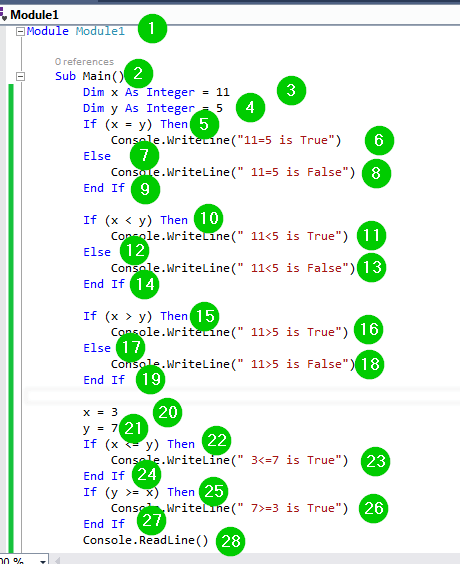Explanation of Code:

1. Creating a module named Module1.
2. Creating the main sub-procedure.
3. Creating an integer variable x with a value of 11.
4. Creating an integer variable y with a value of 5.
5. Checking whether the value of variable x is equal to the value of variable y. We have the If…Then conditional statements.
6. Printing some text on the console if the above condition is True.
7. The Else part to execute if the above condition is False, that is, if x isn’t equal to y.
8. Printing some text on the console if the above Else part executes.
9. Ending the If condition.
10. Checking whether the value of variable x is less than that of variable y.
11. Printing some text on the console if the above condition is true.
12. The Else part to execute if the above condition is False, that is, if the value of variable x is not less than the value of variable y.
13. Printing some text on the console if the above Else part executes.
14. Ending the If condition.
15. Checking whether the value of variable x is greater than that of variable y.
16. Printing some text on the console if the above condition is true.
17. The Else part of executing if the above condition is False, that is, if the value of variable x is not greater than the value of variable y.
18. Printing some text on the console if the above Else part executes.
19. Ending the If condition.
20. Assigning a new value to variable x, that is, from 11 to 3.
21. Assigning a new value to variable y, that is, from 5 to 7.
22. Checking whether the value of variable x is less than or equal to that of variable y.
23. Printing some text on the console if the above condition is true.
24. Ending the If condition.
25. Checking whether the value of variable x is greater than or equal to that of variable y.
26. Printing some text on the console if the above condition is true.
27. Ending the If condition.
28. To exit the console when the user presses any key.

## Logical/Bitwise Operators

These operators help us in making logical decisions.

They include:

 Logical/ Bite wise Operator Descriptions And known as the logical/bitwise AND. Only true when both conditions are true. Or known as the logical/bitwise OR. True when any of the conditions is true. Not The logical/bitwise NOT. To reverse operand’s logical state. If true, the condition becomes False and vice versa. Xor bitwise Logical Exclusive OR operator. Returns False if expressions are all True or False. Otherwise, it returns True. AndAlso It is also known as the logical AND operator. Only works with Boolean data by performing short-circuiting. OrElse It is also known as the logical OR operator. Only works with Boolean data by performing short-circuiting. IsFalse Determines whether expression evaluates to False. IsTrue Determines whether expression evaluates to True.

Let us demonstrate how to use these operators using an example:

Step 1) Create a new console application. If you don’t know how to do it, visit our previous tutorial on Data Types and Variables.

Step 2) Add the following code:

```Module Module1

Sub Main()

Dim var_w As Boolean = True
Dim var_x As Boolean = True
Dim var_y As Integer = 5
Dim var_z As Integer = 20

If (var_w And var_x) Then
Console.WriteLine("var_w And var_x - is true")
End If
If (var_w Or var_x) Then
Console.WriteLine("var_w Or var_x - is true")
End If
If (var_w Xor var_x) Then
Console.WriteLine("var_w Xor var_x - is true")
End If
If (var_y And var_z) Then
Console.WriteLine("var_y And var_z - is true")
End If
If (var_y Or var_z) Then
Console.WriteLine("var_y Or var_z - is true")
End If
'Only logical operators
If (var_w AndAlso var_x) Then
Console.WriteLine("var_w AndAlso var_x - is true")
End If
If (var_w OrElse var_x) Then
Console.WriteLine("var_w OrElse var_x - is true")
End If
var_w = False
var_x = True
If (var_w And var_x) Then
Console.WriteLine("var_w And var_x - is true")
Else
Console.WriteLine("var_w And var_x - is not true")
End If
If (Not (var_w And var_x)) Then
Console.WriteLine("var_w And var_x - is true")
End If
End Sub
End Module
```

Step 3) Run the code by clicking the Start button from the toolbar. You will get the following window: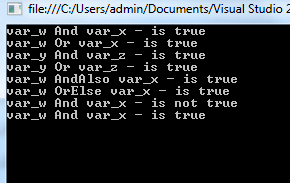Here are screenshots of the above code: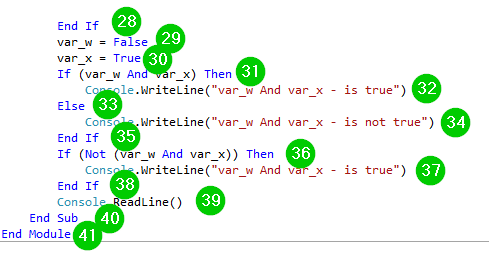Explanation of Code:

1. Creating a module named Module1.
2. Creating the main sub-procedure.
3. Declaring a Boolean variable var_w with a value of True.
4. Declaring a Boolean variable var_x with a value of True.
5. Declaring an integer variable var_y with a value of 5.
6. Declaring an integer variable var_z with a value of 20.
7. Performing And operation on values of variable var_w and var_x. We have used the If…Then condition to take action based on the result of the operation.
8. Text to print on the console if the result of the above operation is True.
9. Ending the If statement.
10. Performing Or operation on values of variable var_w and var_x. We have used the If…Then condition to take action based on the result of the operation.
11. Text to print on the console if the result of the above operation is True.
12. Ending the If statement.
13. Performing Xor operation on values of variable var_w and var_x. We have used the If…Then condition to take action based on the result of the operation.
14. Text to print on the console if the result of the above operation is True.
15. Ending the If statement.
16. Performing And operation on values of variable var_y and var_z. We have used the If…Then condition to take action based on the result of the operation.
17. Text to print on the console if the result of the above operation is True.
18. Ending the If statement.
19. Performing Or operation on values of variable var_y and var_z. We have used the If…Then condition to take action based on the result of the operation.
20. Text to print on the console if the result of the above operation is True.
21. Ending the If statement.
22. A comment. The compiler will skip this.
23. Performing AndAlso operation on values of variable var_w and var_x. We have used the If…Then condition to take action based on the result of the operation.
24. Text to print on the console if the result of the above operation is True.
25. Ending the If statement.
26. Performing OrElso operation on values of variable var_w and var_x. We have used the If…Then condition to take action based on the result of the operation.
27. Text to print on the console if the result of the above operation is True.
28. Ending the If statement.
29. Changing the value of variable w from true to False.
30. The value of variable var_x will remain to be True.
31. Performing And operation on values of variables var_w and var_x. We have used the If…Then condition to take action based on the result of the operation.
32. Text to print on the console if the result of the above operation is True.
33. Else part to be executed if the above If the condition is not True.
34. Text to print on the console if the result of the above If the operation is False. Then it is under the Else statement.
35. Ending the If statement.
36. Performing And operation on values of variables var_w and var_x then reversing the result using the Not operator. We have used the If…Then condition to take action based on the result of the operation.
37. Text to print on the console if the result of the above operation is True.
38. Ending the If statement.
39. Accept input from the user via the keyboard.

## Bit Shift Operators

These operators are used for performing shift operations on binary values.

 Bit-shit operatiors Details And Known as the Bitwise AND Operator. It copies a bit to result if it is found in both operands. Or Known as the Binary OR Operator. It copies a bit if found in either operand. Xor The Binary XOR Operator. For copying a bit if set in one of the operands rather than both. Not It is known as the Binary Ones Complement Operator. It is a unary operator that ‘flips’ the bits.

Let us demonstrate how to use these operators using an example:

Step 1) Create a new console application. If you don’t know how to do it, visit our previous tutorial on Data Types and Variables.

Step 2) Add the following code:

```Module Module1

Sub Main()

Dim w As Integer = 50
Dim x As Integer = 11
Dim y As Integer = 0
y = w And x
Console.WriteLine("y = w And x is {0}", y)
y = w Or x

Console.WriteLine("y = w Or x is {0}", y)
y = w Xor x

Console.WriteLine("y = w Xor x  is {0}", y)
y = Not w

Console.WriteLine("y = Not w is {0}", y)

End Sub
End Module
```

Step 3) Run the code by clicking the Start button. You should get the following window:Here is a screenshot of the code: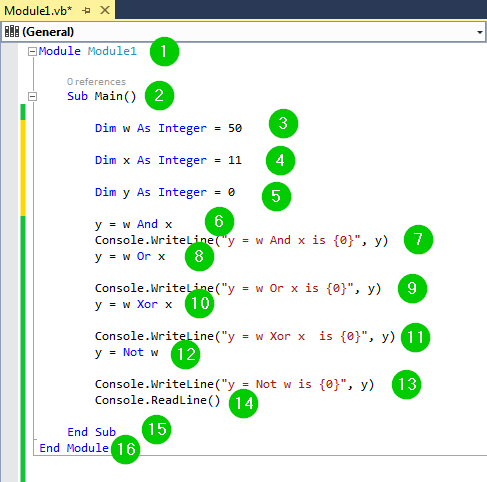Explanation of Code:

1. Creating a module named Module1.
2. Creating the main sub-procedure.
3. Creating an integer variable w with a value of 50.
4. Creating an integer variable x with a value of 11.
5. Creating an integer variable y with a value of 0.
6. Applying the bitwise And operator to the values of variables w and x and assigning the result to variable y.
7. Printing some text and the result of the above operation on the console.
8. Applying the bitwise Or operator to the values of variables w and x and assigning the result to variable y.
9. Printing some text and the result of the above operation on the console.
10. Applying the bitwise Xor operator to the values of variables w and x and assigning the result to variable y.
11. Printing some text and the result of the above operation on the console.
12. Applying the bitwise Not operator to the value of variable w and assigning the result to variable y.
13. Printing some text and the result of the above operation on the console.
14. Pause the console to wait for user input via the keyboard.
15. End the sub-procedure.
16. End the module.

## Assignment Operators

 Assignment Operator Details = the simple assignment operator. It will assign values from the left side operands to the right side operands. += known as the Add AND assignment operator. It will add the right operand to the left operand. Then the result will be assigned to the left operand. = known as the Subtract AND operator. It will subtract the right operand from left operand and assign the result to the left operand. *= : known as the Multiply AND operator. It will subtract the right operand from left operand and assign the result to the left operand.

Let us demonstrate how to use these operators using an example:

Step 1) Create a new console application. If you don’t know how to do it, visit our previous tutorial on Data Types and Variables.

Step 2) Add the following code:

```Module Module1

Sub Main()

Dim x As Integer = 5

Dim y As Integer

y = x
Console.WriteLine(" y = x gives y = {0}", y)

y += x
Console.WriteLine(" y += x gives y = {0}", y)

y -= x
Console.WriteLine(" y -= x gives y = {0}", y)

y *= x
Console.WriteLine(" y *= x gives y = {0}", y)

End Sub
End Module
```

Step 3) Now, run the code by clicking the Start button from the toolbar. You should get the following window: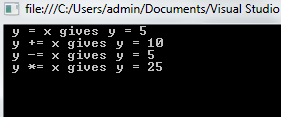The following code has been used:Explanation of Code:

1. Creating a module named Module1.
2. Creating the main sub-procedure.
3. Creating an integer variable x with a value of 5.
4. Creating an integer variable y.
5. Assigning the value of variable x to variable y. The value of variable y now becomes 5.
6. Printing some text and the result of the above operation on the console.
7. Adding the value of variable y to value of variable x, that is, 5 + 5, then assigning the result to variable y. This gives y = 10.
8. Printing some text and the result of the above operation on the console.
9. Subtracting the value of variable x (5) from the value of variable y (10) and assigning the result to variable y, that is, 10-5.
10. Printing some text and the result of the above operation on the console.
11. Multiplying the value of variable y with the value of variable x and assigning the result to variable y, that is, 5 * 5.
12. Printing some text and the result of the above operation on the console.
13. Pause the console waiting for user input.
14. Ending the sub-procedure.
15. Ending the module.

## Miscellaneous Operators

There are other operators supported by VB.NET. Let us discuss them:

 Miscellaneous Operators Details GetType This operator gives the Type of objects for a specified expression. Function Expression for declaring the code and parameters of a lambdaexpression function.

For example:

Step 1) Create a new console application. If you don’t know how to do it, visit our previous tutorial on Data Types and Variables.

Step 2) Add the following code:

```Module Module1

Sub Main()

Dim x As Integer = 5
Console.WriteLine(GetType(Integer).ToString())
Console.WriteLine(GetType(String).ToString())
Console.WriteLine(GetType(Double).ToString())

Dim trippleValue = Function(val As Integer) val * 3
Console.WriteLine(trippleValue(2))
Console.WriteLine(If(x >= 0, "Positive", "Negative"))

End Sub
End Module
```

Step 3) Run the code by clicking the Start button on the toolbar. You should get the following window:We have used the following code:Explanation of Code:

1. Creating a module named Module1.
2. Creating the main sub-procedure.
3. Declaring an integer variable x with a value of 5.
4. Getting the Type object of Integer data type and converting the result to a string by calling the ToString() method and print it on the console.
5. Getting the Type object of String data type and converting the result to a string by calling the ToString() method and print it on a console.
6. Getting the Type object of Double data type and converting the result to a string by calling the ToString() method and printing it on the console.
7. Declaring a lambda function and assigning it to the variable triple value. The lambda function takes an integer parameter named val and multiplies it by 3.
8. Calling the lambda function and passing to it an argument of 2. It will triple this value to give 6.
9. Checking whether the value of variable x is positive or negative. If greater than or equal to 0, it will print Positive, otherwise, Negative.
10. Pause the console waiting for user input.
11. Ending the sub-procedure.
12. Ending the module.

## Summary

• VB.NET supports the use of operators to perform arithmetic, logical, and comparison operations.
• Operators are divided into various categories.
• Operators operate on operands.

#### Top Courses